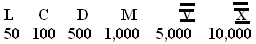Name: ___________________Date:___________________

 Email us to get an instant 20% discount on highly effective K-12 Math & English kwizNET Programs!

### Grade 3 - Mathematics13.4 Roman Numbers - 3

 Rules for writing Roman numbersExample: What is the modern equivalent of LX? Answer: 60 Directions: Write the corresponding Roman numbers. Also write at least 10 examples of your own.
 Q 1: What is the modern equivalent of XXVII?Answer: Q 2: What is the modern equivalent of Roman number D? Answer: Q 3: What is the modern equivalent of XXXIII?Answer: Q 4: What is the modern equivalent of DIV?Answer: Q 5: What is the modern equivalent of LXXV?Answer: Q 6: What is the modern equivalent of Roman number IX?Answer: Q 7: What is the modern equivalent of DX?Answer: Q 8: What is the modern equivalent of Roman number X? Answer: Question 9: This question is available to subscribers only! Question 10: This question is available to subscribers only!

#### Subscription to kwizNET Learning System costs less than \$1 per month & offers the following benefits:

• Unrestricted access to grade appropriate lessons, quizzes, & printable worksheets
• Instant scoring of online quizzes
• Progress tracking and award certificates to keep your student motivated
• Unlimited practice with auto-generated 'WIZ MATH' quizzes